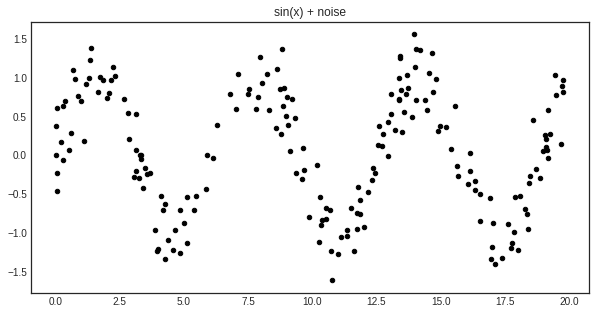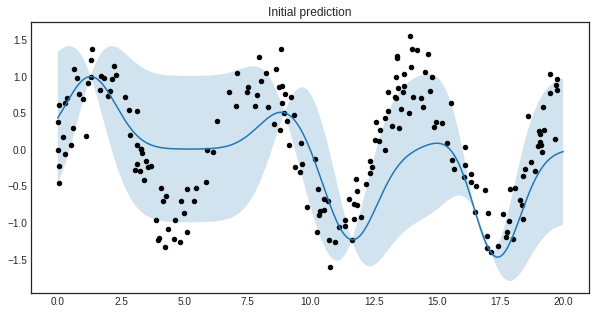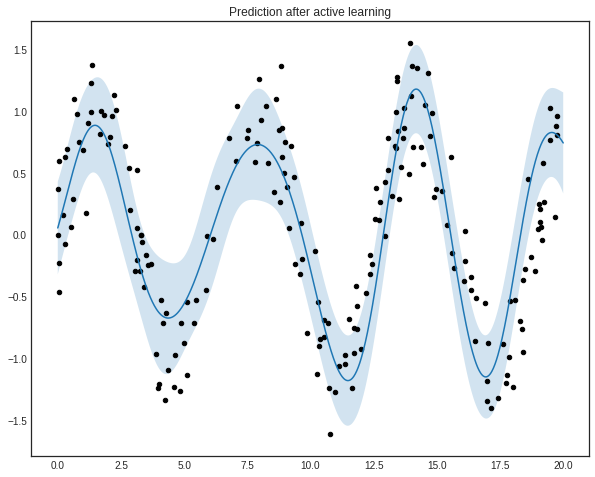# Active regression¶

In this example, we are going to demonstrate how can the ActiveLearner be used for active regression using Gaussian processes. Since Gaussian processes provide a way to quantify uncertainty of the predictions as the covariance function of the process, they can be used in an active learning setting.

:

import numpy as np
import matplotlib.pyplot as plt
from sklearn.gaussian_process import GaussianProcessRegressor
from sklearn.gaussian_process.kernels import WhiteKernel, RBF
from modAL.models import ActiveLearner

%matplotlib inline


## The dataset¶

For this example, we shall try to learn the noisy sine function:

:

X = np.random.choice(np.linspace(0, 20, 10000), size=200, replace=False).reshape(-1, 1)
y = np.sin(X) + np.random.normal(scale=0.3, size=X.shape)

:

with plt.style.context('seaborn-white'):
plt.figure(figsize=(10, 5))
plt.scatter(X, y, c='k', s=20)
plt.title('sin(x) + noise')
plt.show()## Uncertainty measure and query strategy for Gaussian processes¶

For active learning, we shall define a custom query strategy tailored to Gaussian processes. More information on how to write your custom query strategies can be found at the page Extending modAL. In a nutshell, a query stategy in modAL is a function taking (at least) two arguments (an estimator object and a pool of examples), outputting the index of the queried instance and the instance itself. In our case, the arguments are regressor and X.

:

def GP_regression_std(regressor, X):
_, std = regressor.predict(X, return_std=True)
query_idx = np.argmax(std)
return query_idx, X[query_idx]


## Active learning¶

Initializing the active learner is as simple as always.

:

n_initial = 5
initial_idx = np.random.choice(range(len(X)), size=n_initial, replace=False)
X_training, y_training = X[initial_idx], y[initial_idx]

kernel = RBF(length_scale=1.0, length_scale_bounds=(1e-2, 1e3)) \
+ WhiteKernel(noise_level=1, noise_level_bounds=(1e-10, 1e+1))

regressor = ActiveLearner(
estimator=GaussianProcessRegressor(kernel=kernel),
query_strategy=GP_regression_std,
X_training=X_training.reshape(-1, 1), y_training=y_training.reshape(-1, 1)
)


The initial regressor is not very accurate.

:

X_grid = np.linspace(0, 20, 1000)
y_pred, y_std = regressor.predict(X_grid.reshape(-1, 1), return_std=True)
y_pred, y_std = y_pred.ravel(), y_std.ravel()

:

with plt.style.context('seaborn-white'):
plt.figure(figsize=(10, 5))
plt.plot(X_grid, y_pred)
plt.fill_between(X_grid, y_pred - y_std, y_pred + y_std, alpha=0.2)
plt.scatter(X, y, c='k', s=20)
plt.title('Initial prediction')
plt.show()The blue band enveloping the regressor represents the standard deviation of the Gaussian process at the given point. Now we are ready to do active learning!

:

n_queries = 10
for idx in range(n_queries):
query_idx, query_instance = regressor.query(X)
regressor.teach(X[query_idx].reshape(1, -1), y[query_idx].reshape(1, -1))

:

y_pred_final, y_std_final = regressor.predict(X_grid.reshape(-1, 1), return_std=True)
y_pred_final, y_std_final = y_pred_final.ravel(), y_std_final.ravel()

:

with plt.style.context('seaborn-white'):
plt.figure(figsize=(10, 8))
plt.plot(X_grid, y_pred_final)
plt.fill_between(X_grid, y_pred_final - y_std_final, y_pred_final + y_std_final, alpha=0.2)
plt.scatter(X, y, c='k', s=20)
plt.title('Prediction after active learning')
plt.show()